# Friedman Test for Correlated Samples

This is a non-parametric version of the Repeated Measures ANOVA. The test statistic is: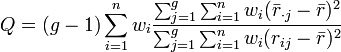$Q =(g-1)\sum_{i=1}^n w_i \frac{ \sum_{j=1}^g\sum_{i=1}^{n} w_i (\bar{r}_{\cdot j} - \bar{r})^2}{\sum_{j=1}^g\sum_{i=1}^{n} w_i (r_{ij} - \bar{r})^2}$

where: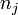$n_j$ is the number of observations in group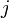$j$ of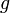$g$groups,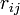$r_{ij}$ is the rank of the$i$th observation from group$j$ where the ranking is computed within observations (e.g., if$g=4$ then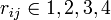$r_{ij} \in {{1,2,3,4}}$), where 1 is assigned to the lowest value and the average rank is used for ties,$n$ is the number of matched samples,$w_i$ is the Calibrated Weight,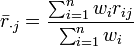$\bar{r}_{\cdot j} = \frac{\sum_{i=1}^n{w_i r_{ij}}}{{\sum_{i=1}^n w_i}}$,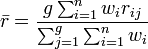$\bar{r} = \frac{g \sum_{i=1}^n w_i r_{ij}}{\sum_{j=1}^g\sum_{i=1}^n w_i}$,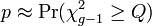$p \approx \Pr(\chi^2_{g-1} \ge Q)$